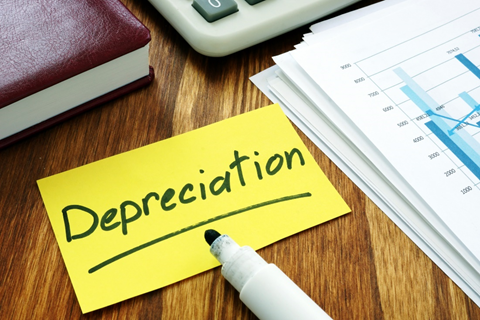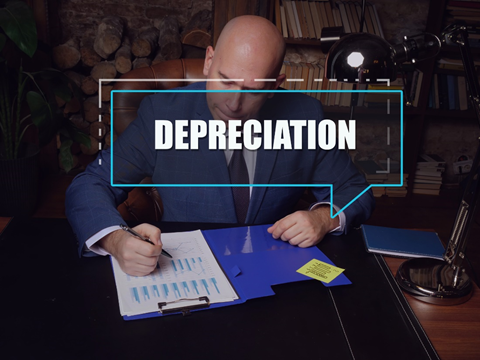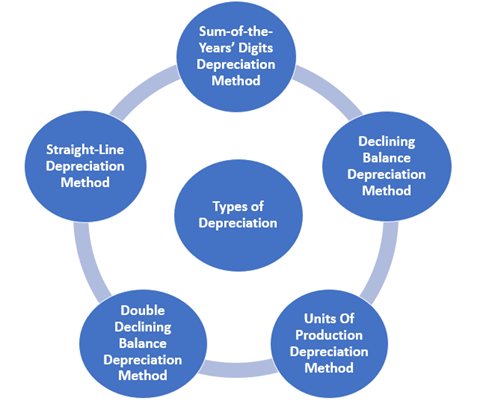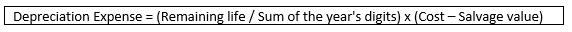# Depreciation

## What do you mean by depreciation?

In accounting, depreciation refers to the cost allocation of both tangible and intangible assets in the accounting statements to periods in which the assets are used. In general, depreciation also refers to reducing the monetary value of assets over time due to their usage, wear, and tear, or because they have become obsolete.

Depreciation gives us a fair idea of how much of an asset's value has been used up. Moreover, depreciating assets enable companies to generate revenue from an asset while expensing a share of its cost every year when the asset is in use. If these assets are not taken into consideration, they can affect a company's profits. Usually, businesses depreciate long-term assets for accounting purposes and tax benefits. For instance, companies can get tax benefits for the expense of an asset. However, the Internal Revenue Service (IRS) has formulated rules regarding when the companies can take a tax deduction. The carrying value of an asset is the difference between its historical cost and the accumulated depreciation.

The carrying value of an asset is maintained in a company's balance sheet.

Summary
• In accounting, depreciation refers to the cost allocation of both tangible and intangible assets in the accounting statements to periods in which the assets are used.
• Depreciation also refers to reducing the monetary value of assets over time due to their usage, wear, and tear.
• Usually, businesses depreciate long-term assets for accounting purposes and tax benefits.

## Do companies have different thresholds to determine when to depreciate an asset?Image Source: © Designer491 | Megapixl.com

In accounting, depreciation usually permits a firm to write off an asset's value over the useful life of an asset. Assets, for example, machinery and equipment, are costly. Rather than recognising the total cost of the asset in a year, depreciation helps a company distribute that cost and earn revenue from it. Depreciation considers the decline in the carrying value of an asset over time. For tangible assets like inventory, equipment, and machinery, carrying cost is "original cost - accumulated depreciation".

On the other hand, for intangible assets such as patents or intellectual property, carrying cost is the difference between the historical cost and the amortisation expense. Thus, the formula is "original cost - amortisation expense." Nevertheless, an asset's salvage value is the carrying value of an asset after all depreciation is completed.

Companies can have their threshold amount determining when to start depreciating tangible assets, also known as fixed assets. For instance, a medium-sized company can set a \$900 threshold, exceeding which depreciates an asset. Whereas a larger company can set a \$12,000 threshold, below which all purchases are expensed quickly.

## Why is depreciation considered a non-cash expense?

Depreciation schedules are prepared by the IRS at regular intervals, enlisting details such as the number of years an asset can be depreciated depending on the class of assets. When an asset is purchased, companies can pay the entire cash outlay initially; however, the expense is spread out and is recorded gradually for financial reporting. This is because assets prove to be beneficial for a company for an extended period. For this reason, depreciation is regarded as a non-cash charge because it does not depict actual cash outflow. Depreciation helps a company in tax deduction because depreciation charge decreases a company's earnings.

## What is the depreciation rate?

The depreciation rate is depicted as a percentage of the total amount that is depreciated every year. For instance, if a firm had \$100,000 as total depreciation over the asset's useful life, the depreciation for the year was \$16,000; the rate would be 16% each year.

## How do companies record depreciation?Image Source: © Rummess | Megapixl.com

When a company buys an asset, it records it as a debit to increase an asset account, which later appears on the balance sheet, and a credit to reduce cash or increase accounts payable. These entries are reflected in a company's balance sheet. Again, though, the entries do not affect the income statement of the company. Both revenues and expenses are mentioned on a company's income statement.

After an accounting cycle, an accountant will record depreciation for all capitalized assets that are not entirely depreciated. The register entry for this depreciation comprises of a debit to depreciation expense and credit to accumulated depreciation. Thus, debit to depreciation expense flows via the income statement, whereas credit to accumulated depreciation is mentioned on the balance sheet.

As discussed above, an asset's carrying value is the difference between its initial cost and the accumulated depreciation. In contrast, the salvage value is the carrying value that remains after all depreciation is complete. It depends on a company as to what it anticipates receiving in replacement for the asset whose life has ended. Thus, an asset's estimated salvage value is an essential component for calculating depreciation.

## Explain depreciation with the help of an example.

If a firm purchases machinery for \$60,000, it can make either expense the entire cost of the asset in the first year of the purchase or write off its value over the asset's 20-year useful life. Business owners choose to expense only a portion of the cost involved in purchasing the said asset and not the total cost because it boosts their income.

Besides, the firm can scrap the machinery for \$20,000 after the completion of its useful life. This would suggest the salvage value of the machinery to be \$20,000. Based on these variables, one can calculate depreciation expense as the difference between the asset's initial cost and its salvage value, divided by the asset's life expectancy. The method will be (\$60,000 - \$20,000) / 20, which will come as \$2,000 of depreciation expense every year.

The above example means that the accountant does not need to expense the entire \$60,000 in the first year, although the firm had paid that amount in cash. Instead, the firm only needs to expense \$2,000 against the net income. Every passing year, the firm will expense \$2,000 yearly until the asset reaches \$20,000 salvage value in 20 years.

## Discuss the most common types of depreciation?Straight-line depreciation method

Straight-line depreciation is usually the most common method to record depreciation. This method includes equal or similar depreciation expense for every year throughout the useful life of an asset unless the said asset reaches its salvage value.

Declining balance depreciation method

The declining balance method is best suited for those assets that quickly lose their values. An example can be cell phones and computers. This method is just the opposite of the straight-line depreciation method. Instead, this method subtracts the salvage value from the asset's cost, divided by the asset's useful life.

The formula to calculate declining balance depreciation is:Double declining balance (DDB) depreciation

The double-declining balance (DDB) depreciation method is mainly used in business accounting to account for the expense of long-term assets. This method records more considerable depreciation expenses during the initial years of an asset's life expectancy and smaller ones in later years. Besides, this method depreciates assets twice as fast as compared to the declining balance method.

Sum-of-the-Years'-Digits (SYD) depreciation method

The depreciation formula for the sum-of-the-years-digits method is:The sum-of-the-years-digits depreciation method provides higher depreciation costs in the starting years and lower depreciation charges during the later years.

Units of production depreciation method

This method requires the total units that will be produced during the useful life of an asset. Thus, this method depreciates assets depending on the total number of hours used or the total number of units produced using the asset during its useful life.

##### Top ASX Listed Companies

Definition:
We use cookies to ensure that we give you the best experience on our website. If you continue to use this site we will assume that you are happy with it. OK